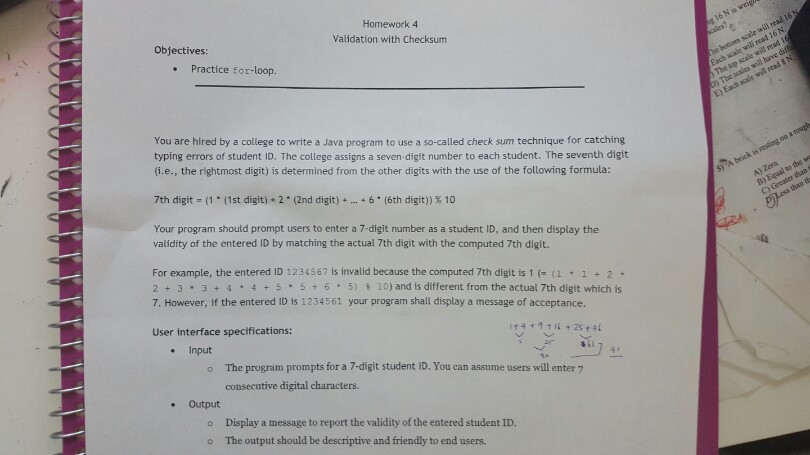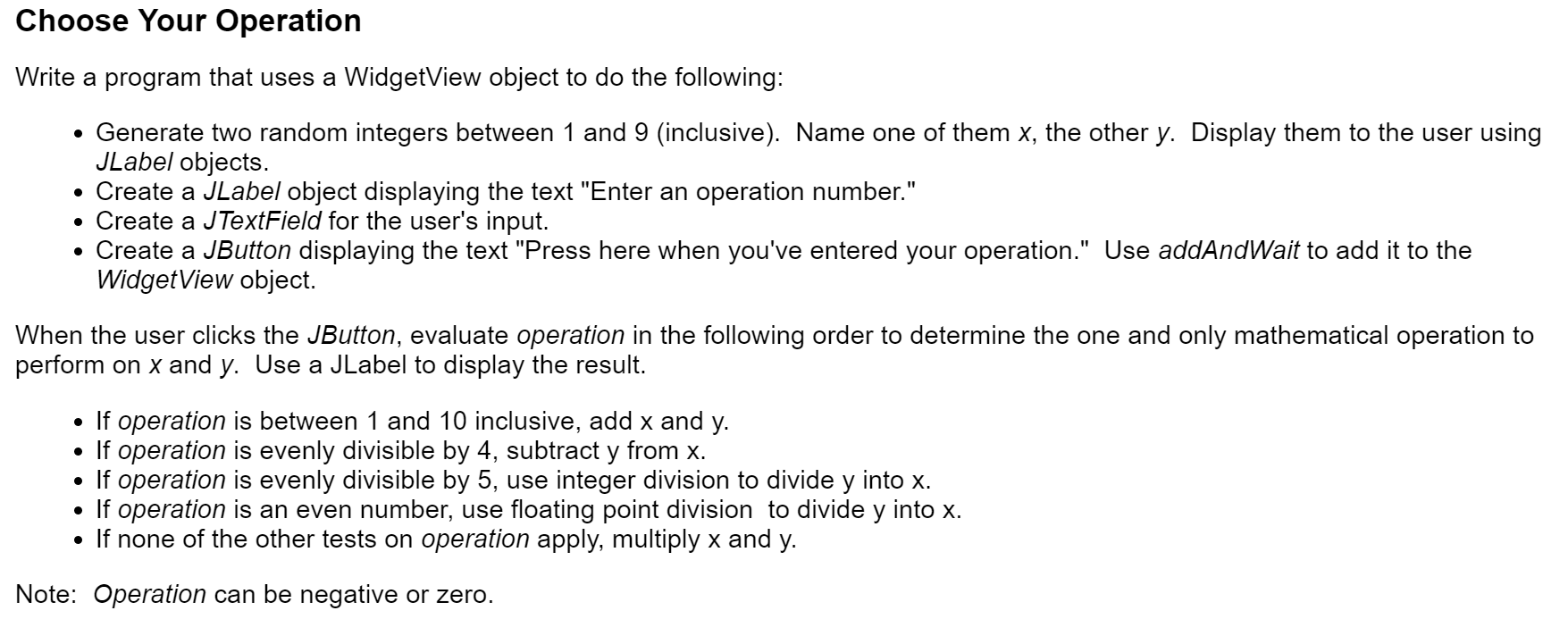Question

# Homework: Write a sample program in Java to compute the number of chocolate spheres stacked in...

Homework:

Write a sample program in Java to compute the number of chocolate spheres stacked in a triangular pyramid within x triangular layers. n(x) is the number of balls required to make x layers.

The value of x is entered by users.

(Try for x = 4, x =5.)

//Formula//

n(x) = 1/6 * x^3 + 1/2 * x^2 + 1/3 x

*No if, no loops. Use Java, and be basic so I can understand your coding.

Thanks for the question, here is the java code with comments so that you can underatnd and learn.

Let me know if you need any help with any other java questions

thank you !

=====================================================================

import java.io.IOException;

public class BallPyramid {

public static void main(String[] args) {

// create a BufferedReader object to read user input that is the value of x

System.out.print("Enter the number of layers: ");
try {
// read user input in the below line and convert to integer number

// calculate the number of balls needed in the below line of code

int ballsNeeded = (layers*layers*layers)/6 +(layers*layers)/2 + layers/3;
// print the balls needed count

System.out.println("Total Balls Required: "+ballsNeeded);

} catch (IOException e) { // incase there are any errors reading the input
System.out.println("Error: Input error");
}

}
}

=====================================================================

#### Earn Coins

Coins can be redeemed for fabulous gifts.

Similar Homework Help Questions
• ### Homework description::::: Write JAVA program with following description. Sample output with code will be helful... A...

Homework description::::: Write JAVA program with following description. Sample output with code will be helful... A compiler must examine tokens in a program and decide whether they are reserved words in the Java language, or identifiers defined by the user. Design a program that reads a Java program and makes a list of all the identifiers along with the number of occurrences of each identifier in the source code. To do this, you should make use of a dictionary. The...

• ### Write a JAVA program that plays a number guessing game with the user. A sample run...

Write a JAVA program that plays a number guessing game with the user. A sample run for the game follows. User input is shown in boldface in the sample run. Welcome to the game of Guess It! I will choose a number between 1 and 100. You will try to guess that number. If your guess wrong, I will tell you if you guessed too high or too low. You have 6 tries to get the number. OK, I am...

• ### You are hired by a college to write a Java program to use a so-called check...You are hired by a college to write a Java program to use a so-called check sum technique for catching typing errors of student ID. The college assigns a seven-digit number to each student. The seventh digit (i.e., the rightmost digit) is determined from the other digits with the use of the following formula: 7th digit = (1 *(1st digit) * 2 * (2nd digit) * ... * 6 * (6th digit)) % 10 Your program should prompt users to...

• ### Write a java program to print all the powers of 2 below a certain number and...

Write a java program to print all the powers of 2 below a certain number and calculate their sum Requirements: 1. Accept the upper limit from the user as an integer 2. Make sure the sum variable is of the “long” type. You can assume that the test output will be less than LONG MAX. 3. Print all the numbers as a running sum, and finally print their sum. Please try and restrict yourself to loops, selection statements, and calls...

• ### Use Java program Material Covered : Loops & Methods Question 1: Write a program to calculate...

Use Java program Material Covered : Loops & Methods Question 1: Write a program to calculate rectangle area. Some requirements: 1. User Scanner to collect user input for length & width 2. The formula is area = length * width 3. You must implement methods getLength, getWidth, getArea and displayData ▪ getLength – This method should ask the user to enter the rectangle’s length and then return that value as a double ▪ getWidth – This method should ask the...

• ### This is a java homework for my java class. Write a program to perform statistical analysis...

This is a java homework for my java class. Write a program to perform statistical analysis of scores for a class of students.The class may have up to 40 students.There are five quizzes during the term. Each student is identified by a four-digit student ID number. The program is to print the student scores and calculate and print the statistics for each quiz. The output is in the same order as the input; no sorting is needed. The input is...

• ### Write a java calculator program that perform the following operations: 1. function to Addition of two...

Write a java calculator program that perform the following operations: 1. function to Addition of two numbers 2. function to Subtraction of two numbers 3. function to Multiplication of two numbers 4. function to Division of two numbers 5. function to Modulus (a % b) 6. function to Power (a b ) 7. function to Square root of () 8. function to Factorial of a number (n!) 9. function to Log(n) 10. function to Sin(x) 11. function to Absolute value...

• ### JAVA PROBLEM #1: The British have been shelling the Revolutionary forces with a large cannon from...

JAVA PROBLEM #1: The British have been shelling the Revolutionary forces with a large cannon from within their camp. You have been assigned a dangerous reconnaissance mission -- to infiltrate the enemy camp and determine the amount of ammunition available for that cannon. Fortunately for you, the British (being relatively neat and orderly) have stacked the cannonballs into a single pyramid-shaped stack. At the top is a single cannonball resting on a square of 8 cannonballs, which is itself resting...Please help me the JAVA program - Choose Your Operation Write a program that uses a WidgetView object to do the following: Generate two random integers between 1 and 9 (inclusive). Name one of them x, the other y. Display them to the user using JLabel objects. Create a JLabel object displaying the text "Enter an operation number." Create a JTextField for the user's input. Create a JButton displaying the text "Press here when you've entered your operation." Use addAndWait...

• ### (a) Write a program in Java to implement a recursive search function int terSearch(int A[], int...

(a) Write a program in Java to implement a recursive search function int terSearch(int A[], int l, int r, int x) that returns the location of x in a given sorted array of n integers A if x is present, otherwise -1. The terSearch search function, unlike the binary search, must consider two dividing points int d1 = l + (r - l)/3 int d2 = d1 + (r - l)/3 For the first call of your recursive search function...